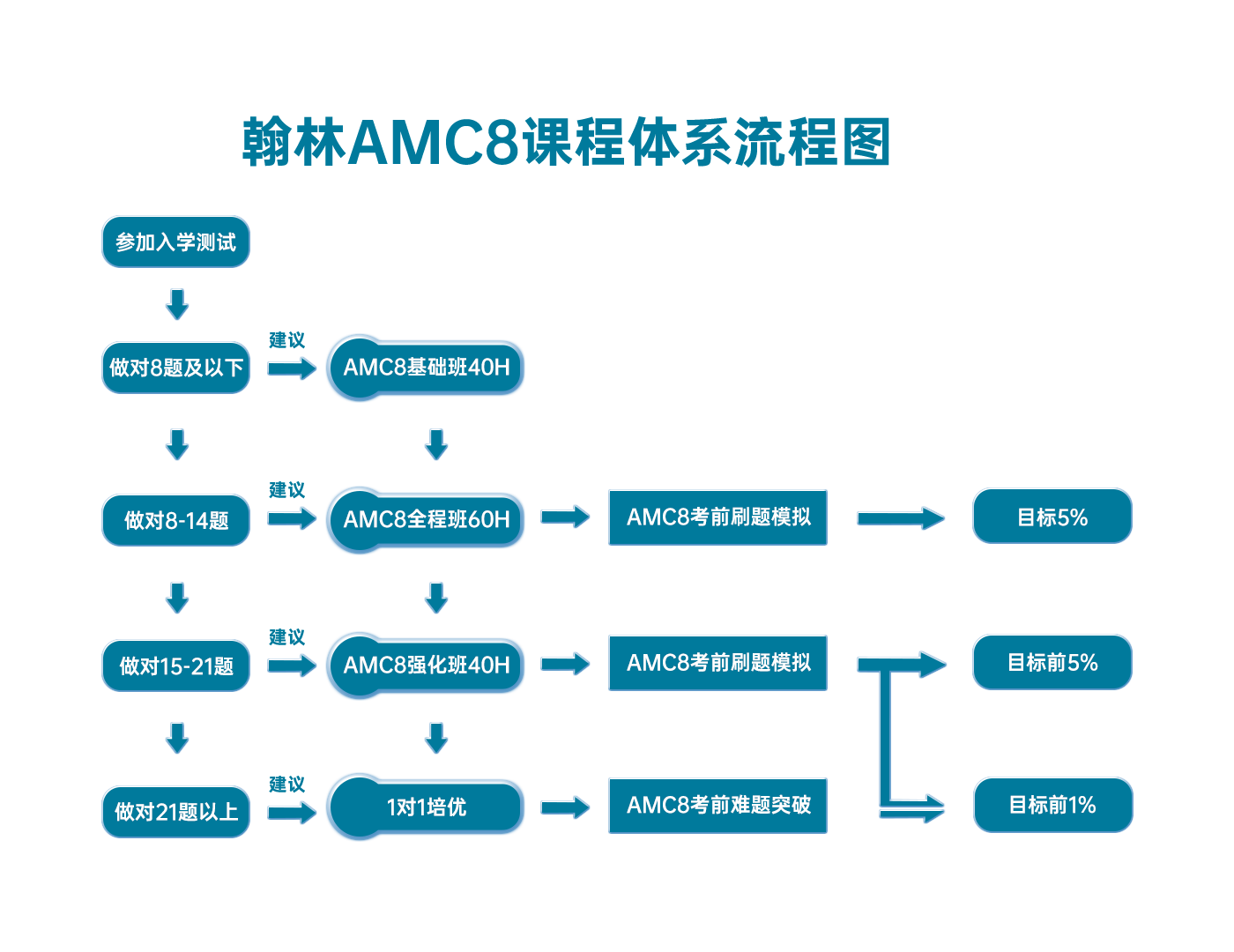# 2016 AMC8 答案

1. It is best to split 11 hours and 5 minutes into 2 parts, one of 11 hours and another of 5 minutes. We know that there is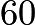minutes in a hour. Therefore, there areminutes in 11 hours. Adding the second part(the 5 minutes) we get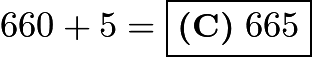.

2.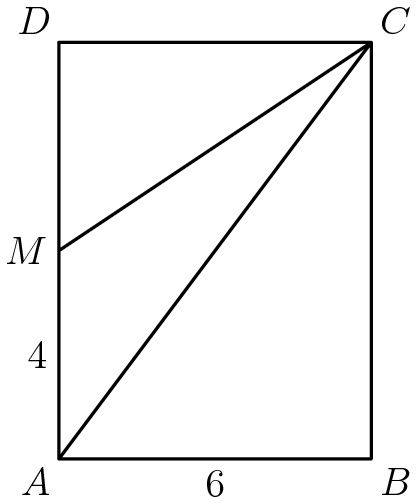### Solution 1

Use the triangle area formula for triangles: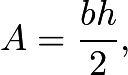where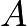is the area,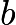is the base, and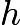is the height. This equation gives us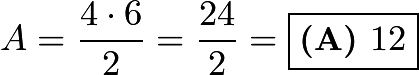.

### Solution 2

A triangle with the same height and base as a rectangle is half of the rectangle's area. This means that a triangle with half of the base of the rectangle and also the same height means its area is one quarter of the rectangle's area. Therefore, we get.

3. We can call the remaining score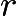. We also know that the average, 70, is equal to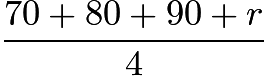. We can use basic algebra to solve for: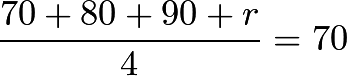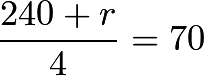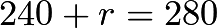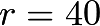giving us the answer of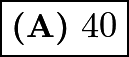.

4.  When Cheenu was a boy, he could run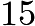miles in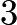hours and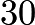minutes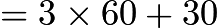minutes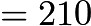minutes, thus running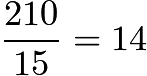minutes per mile. When he is an old man, he can walk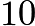miles in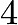hours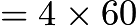minutes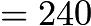minutes, thus walking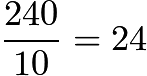minutes per mile. Therefore it takes him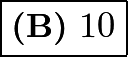minutes longer to walk a mile now compared to when he was a boy.
5. ## Solution 1

For the first three digits, there are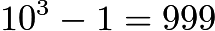combinations since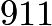is not allowed. For the final digit, any of thenumbers are allowed.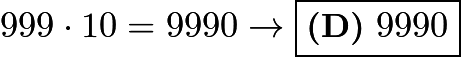## AMC8/AMC10/AMC12/AIME

AMC8火热报名中！### 翰林AMC8课程体系流程图